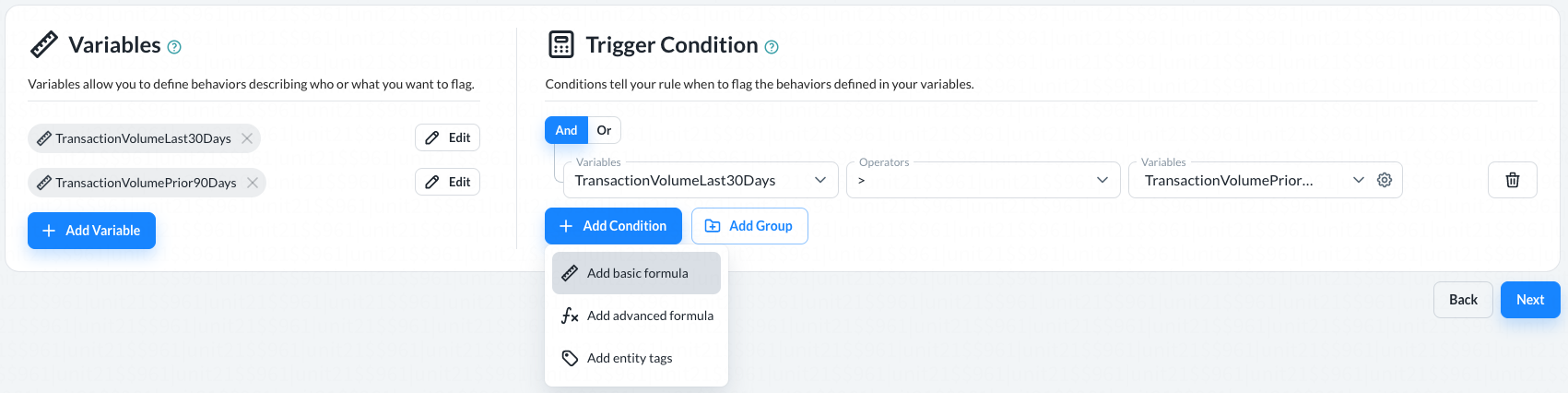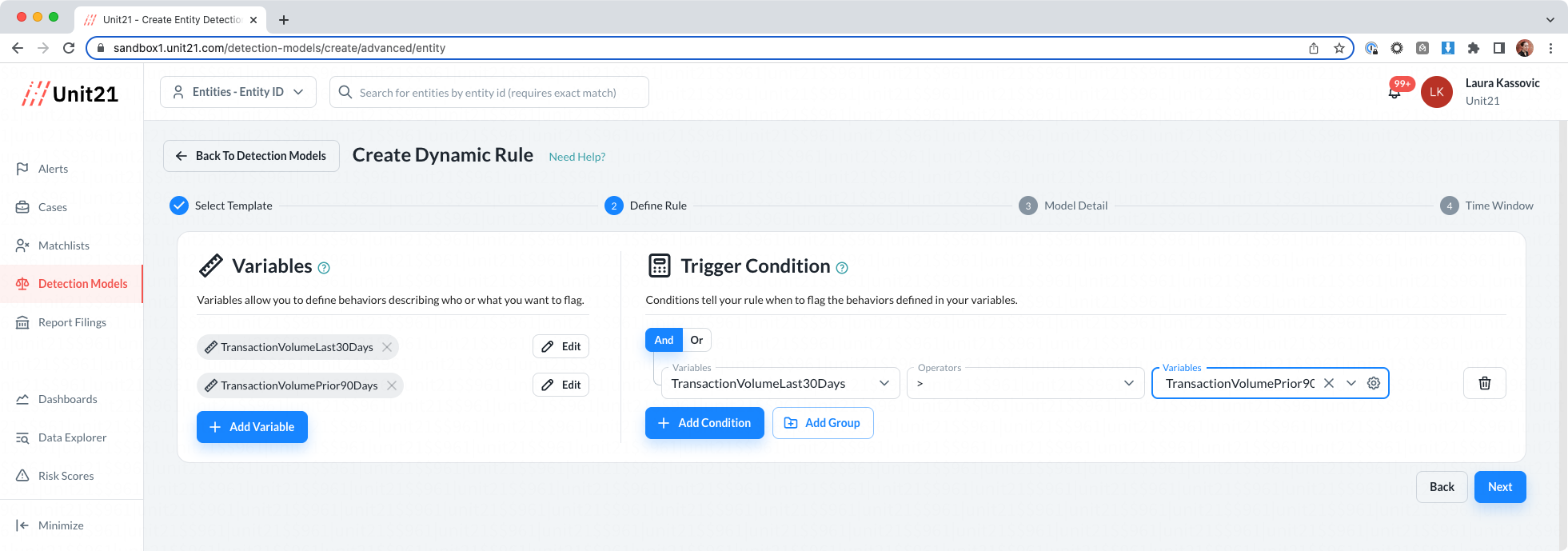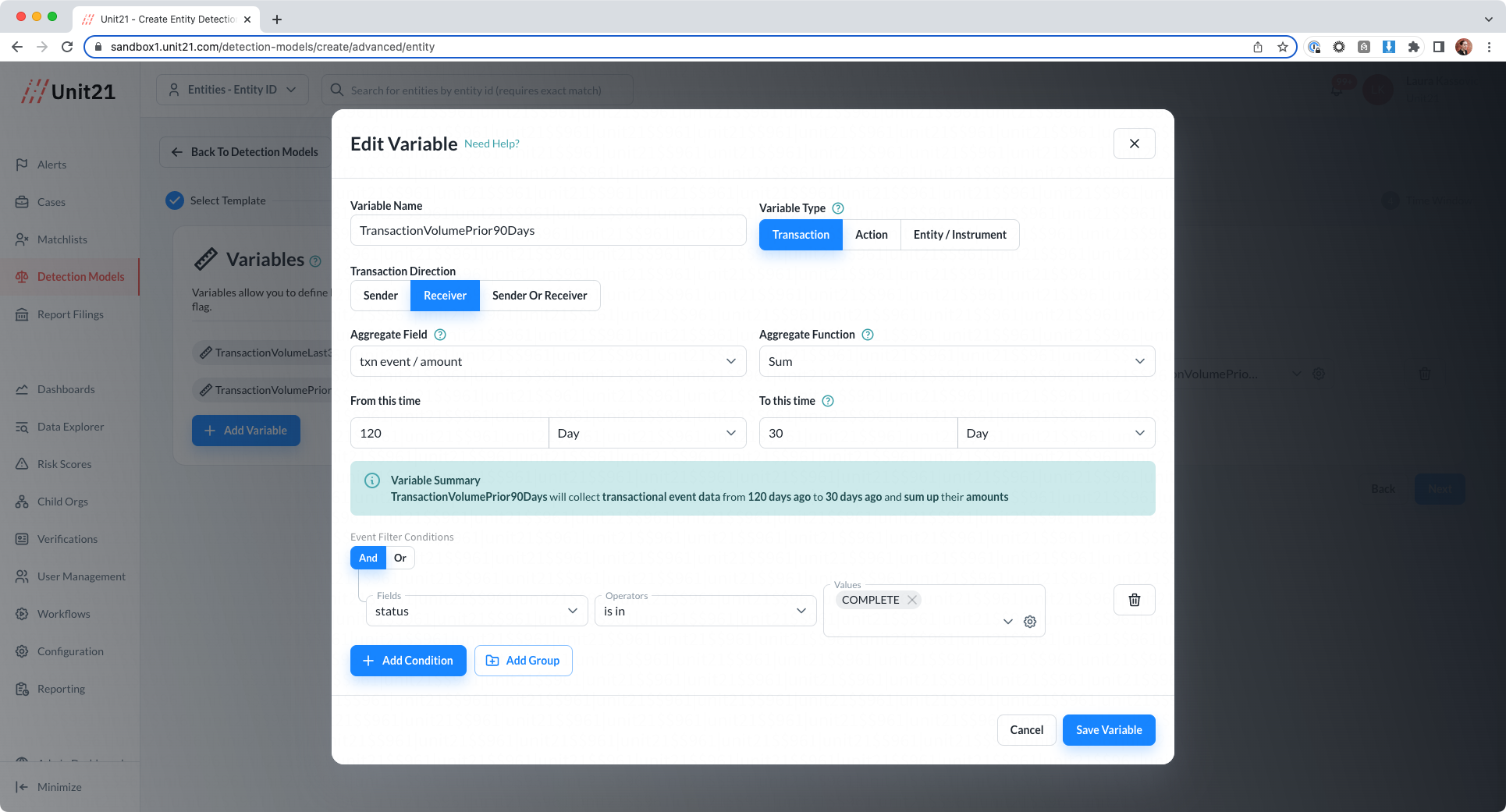# Understanding the Dropdown Builder (Basic Formula)

The dynamic model builder uses drop-downs to apply filters and formulaic logic. This is best used when creating simple rules.By default, the simple logic defaults to a numeric value:

### Numeric OperatorsSymbolName
`!=`Not equal
`=`Equal
`>=`Greater than or equal to
`<=`Less than or equal to
`<`Less than
`>`Greater than
`<=`Less than or equal to
`BETWEEN`Between `value X` and `value Y`

### Logic OperatorsSymbolName
`is in`Equals X,Y, or Z
`is not in`Does not equal X,Y or Z
`contains`Equals any of X,Y or Z
`does not contain`Does not equal any of X,Y or Z
`is equal to`= X
`is not equal to`!= X